``````// 声明一些变量并初始化它们
var a = 5
let b = 'xy'
const c = true

// 分配新值
a = 6
b = b + 'z'
c = false //  类型错误:不可对常量赋值
``````

• JS 原始数据类型的变量声明和赋值
• JavaScript内存模型：调用堆栈和堆
• JS 引用类型的变量声明和赋值
• `let` vs `const`

## JS 原始数据类型的变量声明和赋值

``````let myNumber = 23
``````

1. 为变量(`myNumber`)创建唯一标识符(identifier)。
2. 在内存中分配一个地址(在运行时分配)。
3. 将值 `23` 存储在分配的地址。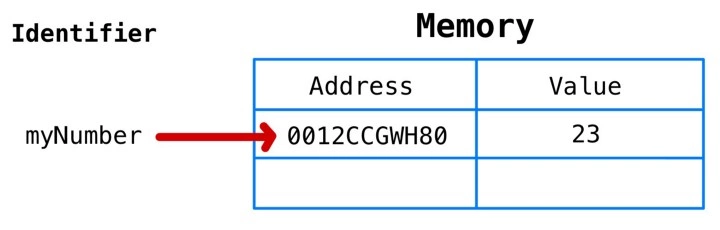``````let newVar = myNumber
``````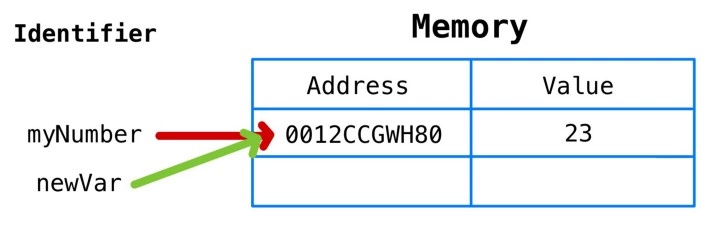``````myNumber = myNumber + 1
``````

`myNumber`的值肯定是 24。但是`newVar`的值是否也为 24 呢？，因为它们指向相同的内存地址？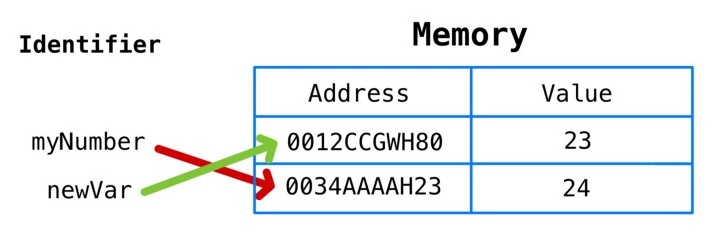``````let myString = 'abc'
myString = myString + 'd'
``````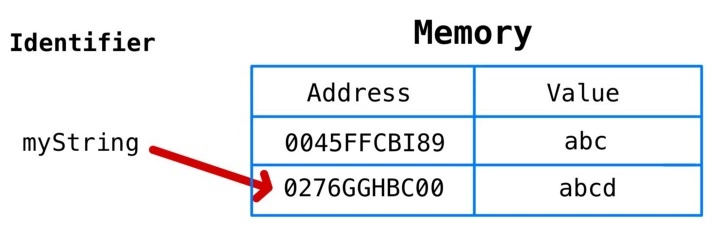## JavaScript 内存模型：调用堆栈和堆

JS 内存模型可以理解为有两个不同的区域:调用堆栈(call stack)堆(heap)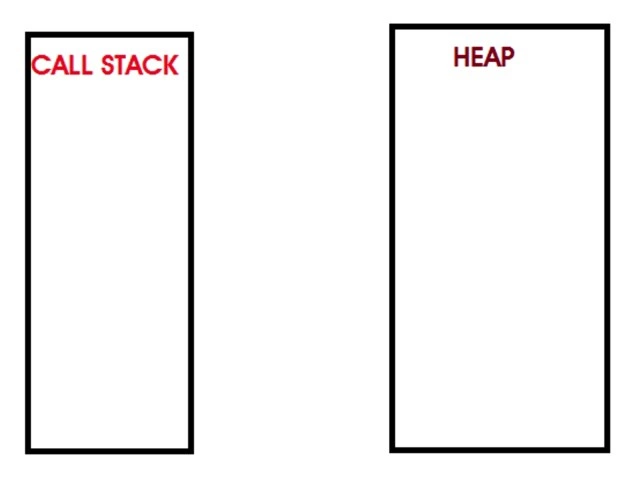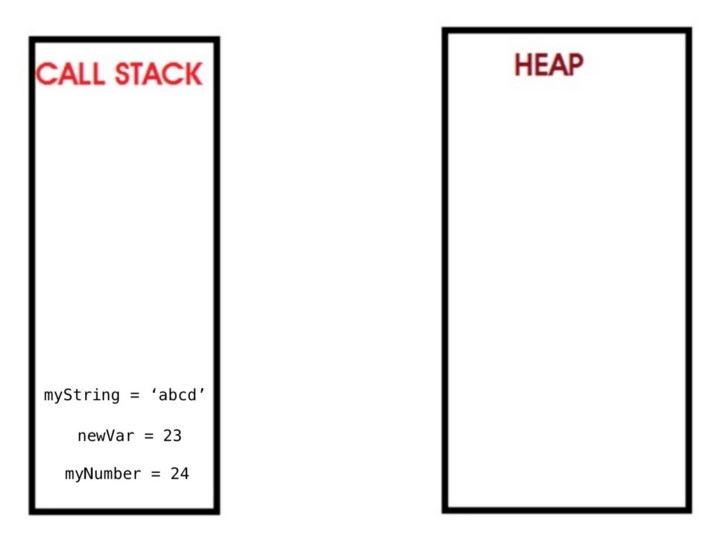## JS 引用类型的变量声明和赋值

``````let myArray = []
``````

1. 为变量创建唯一标识符（“`myArray`”）
2. 在内存中分配一个地址（将在运行时分配）
3. 存储在堆上分配的内存地址的值（将在运行时分配）
4. 堆上的内存地址存储分配的值（空数组[]）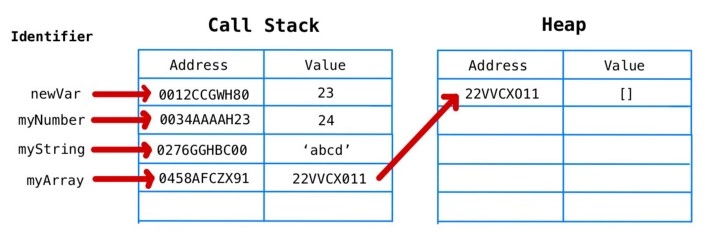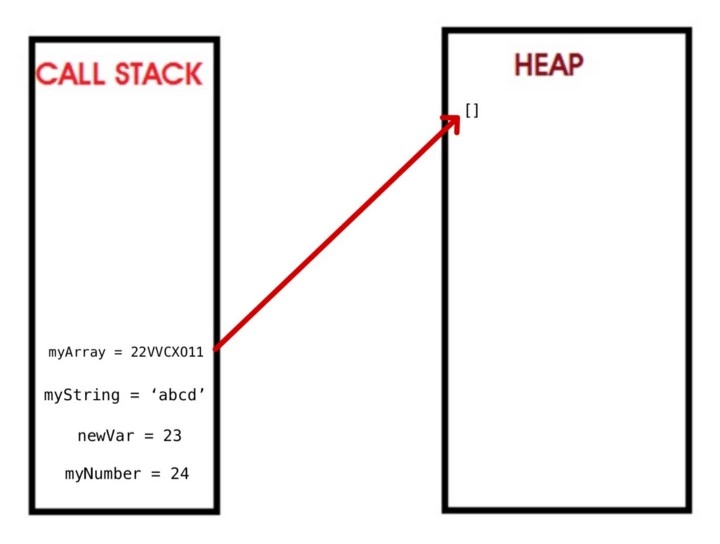``````myArray.push("first")
myArray.push("second")
myArray.push("third")
myArray.push("fourth")
myArray.pop()
``````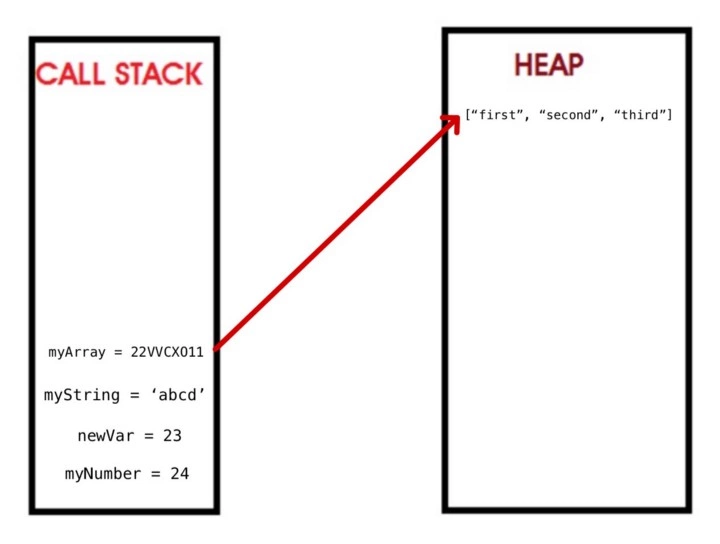## let `vs` const

``````let sum = 0
sum = 1 + 2 + 3 + 4 + 5
let numbers = []
numbers.push(1)
numbers.push(2)
numbers.push(3)
numbers.push(4)
numbers.push(5)
``````

``````const importantID = 489
importantID = 100 // 类型错误:赋值给常量变量
``````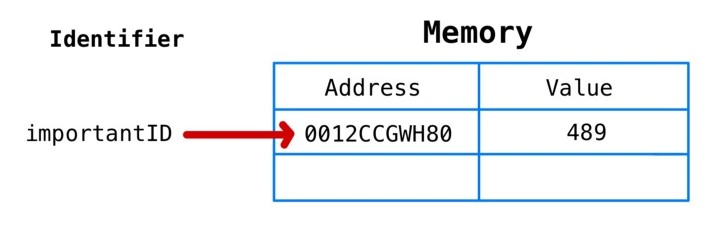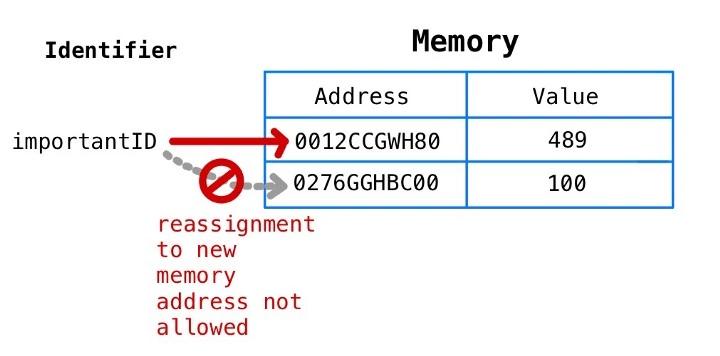``````const myArray = []
``````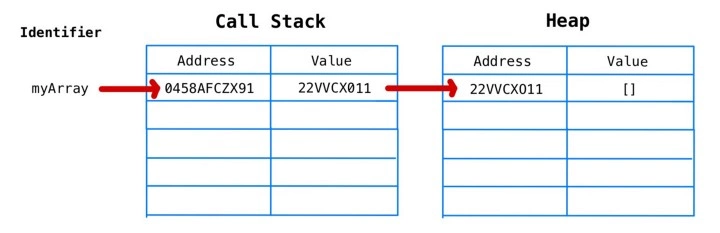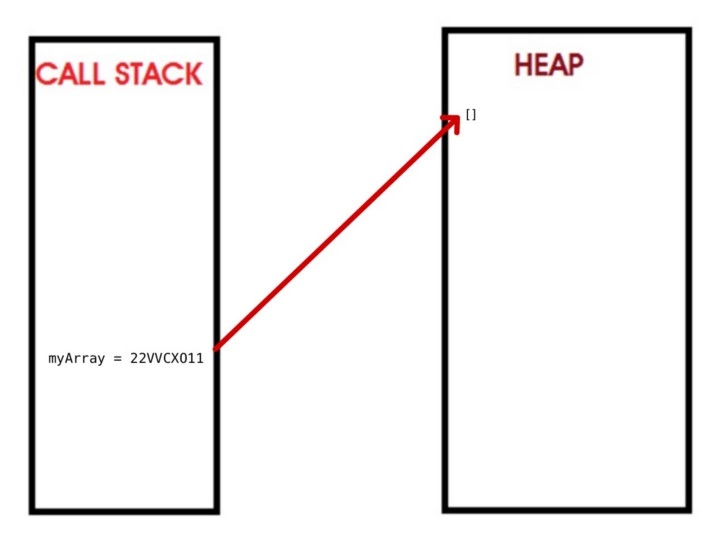``````myArray.push(1)
myArray.push(2)
myArray.push(3)
myArray.push(4)
myArray.push(5)
``````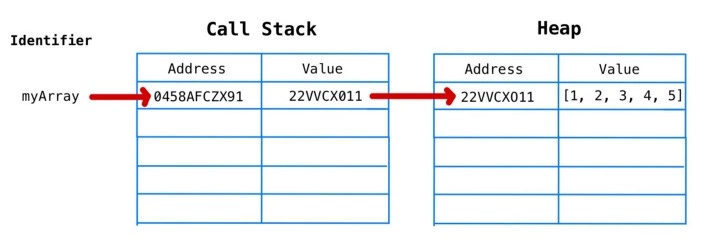`myArray` 仍然等于 `0458AFCZX91`，它的值是另一个内存地址`22VVCX011`，它在堆上有一个数组的值。

``````myArray = 3
``````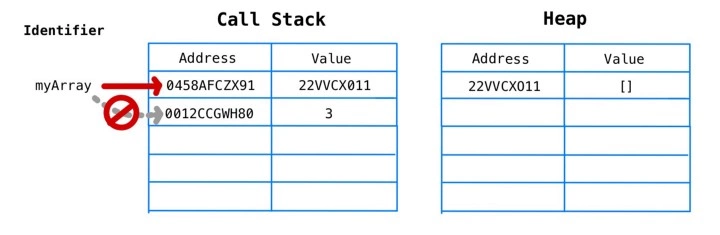``````myArray = ['a']
``````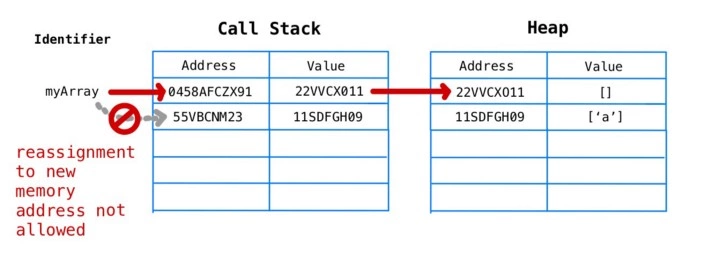``````const myObj = {}
myObj['newKey'] = 'someValue' // 这不会抛出错误
``````

## 为什么这些知识对我们有用呢

JavaScript 是世界上排名第一的编程语言（根据GitHub和Stack Overflow的年度开发人员调查）。 掌握并成为“JS忍者”是我们所有人都渴望成为的人。

1. 先发制人地限制未来的 bug。
2. 使用 `const` 声明的变量必须在声明时初始化，这迫使程序员经常在范围方面更仔细地放置它们。这最终会导致更好的内存管理和性能。
3. 要通过代码与任何可能遇到它的人交流，哪些变量是不可变的(就JS而言)，哪些变量可以重新分配。